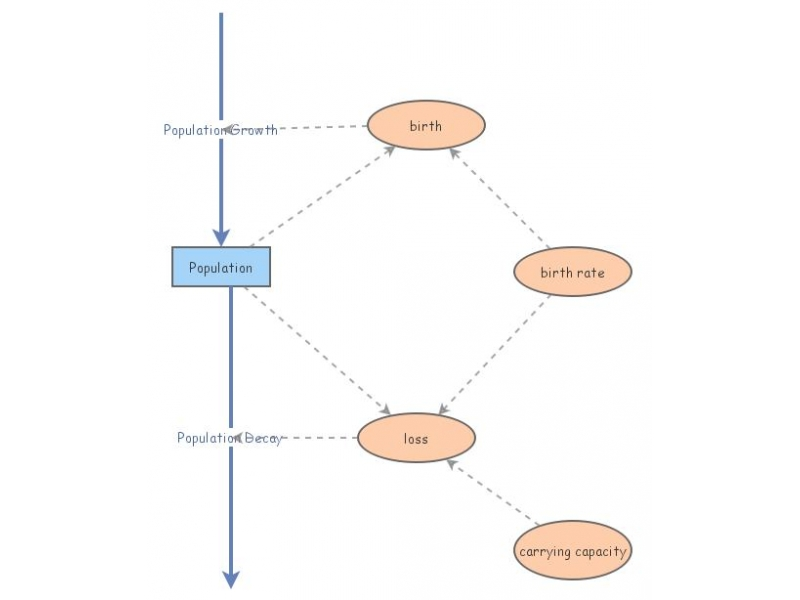Clone of Logistic GrowthThis simple model demonstrates logistic growth.The differential equation looks like

y'(t)=by(t)(1-y(t)/K)

where K is the carrying capacity of the quantity y. Alternatively,

y'(t)=by(t) - b/K*y(t)^2

so the growth term suggests exponential growth, but there is a loss term is of the form b/K y(t) -- loss is proportional to population (crowding).

A comparable Mathematica file is available at
http://www.nku.edu/~longa/classes/2018spring/mat375/mathematica/LogisticGrowth-and-DecayModel.nb